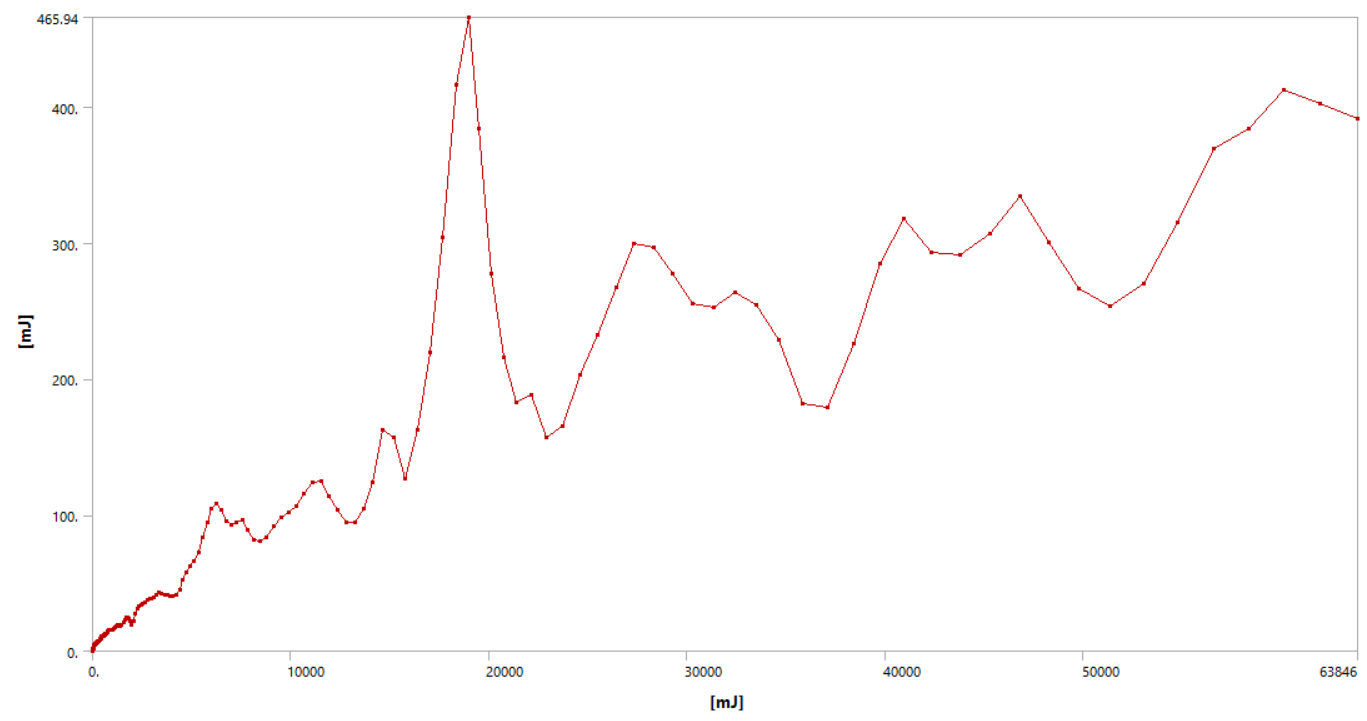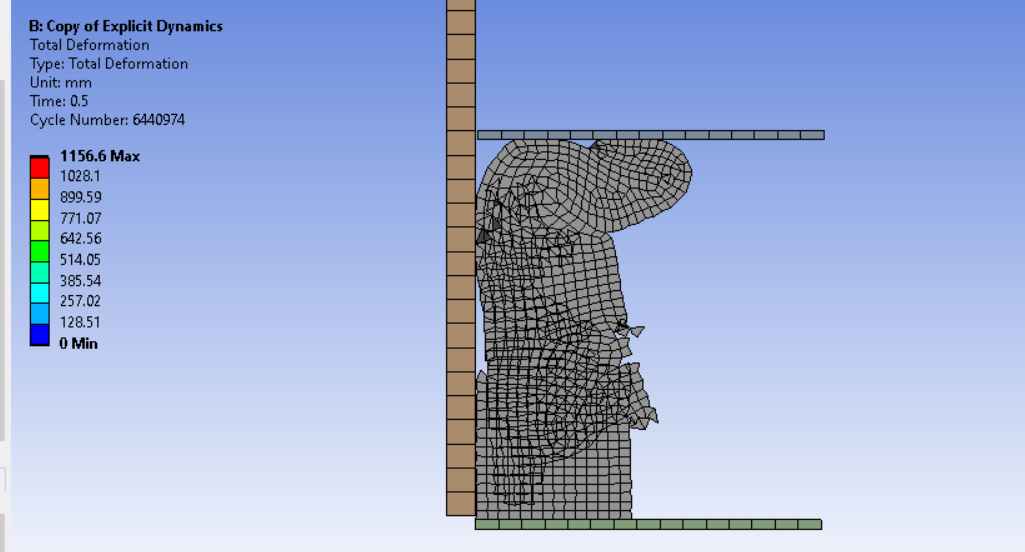Mahdi_171
Subscriber
Dear Peter,nThank you so much. It would have taken me much more time to reach this point. I spent some time reading about the topic, and I found this equation.nThe reference says that the loading velocity should be less than 1% of the wave velocity (estimated by the previous equation) to minimize the inertia effect. I changed the elastomer to one with a higher Young's modulus to increase the wave velocity and see what happens.nI got this plot for the kinetic vs total energy.nI don't know if I can consider this as a quasi-static simulation, so I tried a slower one but with constant velocity, and this happened.nnCould this be a result of high-frequency that you mentioned? The simulation was fine till a point where the elements were crushed like this, but it didn't stop and was completed to the end. The energy plot was better now, with almost zero kinetic energy before the crushing.Another thing I want to know, if possible, how can I view the deformed model in 3D. I tried to do cyclic symmetry, but the option is hidden, and the help section doesn't explain anything about that.n